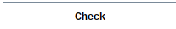Overview - Maple HelpMath AppsIntroduction

Maple's Math Apps and applications provide demonstrations that illustrate various mathematical and scientific concepts. Use this guide to quickly navigate through these math apps and applications. Click an icon to open the corresponding Maple worksheet.Algebra & GeometryBasicsCalculusComputer ScienceDiscrete MathematicsEngineering & ApplicationsFinance & EconomicsFunctions & RelationsGraphingLogic & PuzzlesNatural SciencesProbability & StatisticsReal & Complex NumbersTrigonometryGradeable AppsSee Also